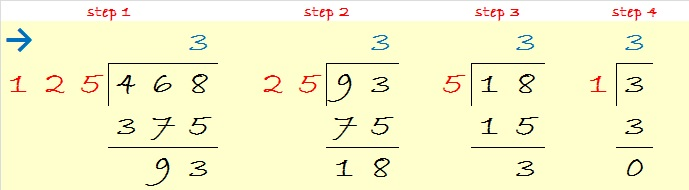# 468 and Level 3

468 is the sum of some consecutive primes. One of my readers posted what those primes are in the comments.

468 is 3333 in base 5. (Thank you OEIS.org for that cool fact.) Here’s proof going from right to left using some easy division problems. (See 3333 at the bottom.)And here’s proof going from left to right using some more difficult division problems. (See 3333 at the top.)—————————————————————————————————

The last two digits of 468 can be evenly divided by 4, so 468 is also divisible by 4.

468 is made from three consecutive even numbers so it is divisible by 3. Since the middle digit of the three consecutive even numbers is divisible by 3, we know that 468 can also be evenly divided by 9.

Let’s use those two facts to simplify the square root of 468 using the modified cake method. If you’re very confident in your ability to divide, you can make a one-layer cake and simply divide 468 by 36 to get 13. Then take the square root of everything on the outside of the cake and multiply them together: √468 = (√36)(√13) = 6√13

Many people will feel more comfortable making a two layer cake by dividing first by 4 and then by 9 as illustrated below:Then to simplify √468, take the square root of everything on the outside of the cake and multiply those square roots together: √468 = (√4)(√9)(√13) = (2 x 3)(√13) = 6√13

You only need to know multiplication facts up to 12 x 12 to solve this factoring puzzle:Print the puzzles or type the solution on this excel file:  12 Factors 2015-04-20

—————————————————————————————————

• 468 is a composite number.
• Prime factorization: 468 = 2 x 2 x 3 x 3 x 13, which can be written 468 = (2^2) x (3^2) x 13
• The exponents in the prime factorization are 2, 2 and 1. Adding one to each and multiplying we get (2 + 1)(2 + 1)(1 + 1) = 3 x 3 x 2 = 18. Therefore 468 has exactly 18 factors.
• Factors of 468: 1, 2, 3, 4, 6, 9, 12, 13, 18, 26, 36, 39, 52, 78, 117, 156, 234, 468
• Factor pairs: 468 = 1 x 468, 2 x 234, 3 x 156, 4 x 117, 6 x 78, 9 x 52, 12 x 39, 13 x 36 or 18 x 26
• Taking the factor pair with the largest square number factor, we get √468 = (√36)(√13) = 6√13 ≈ 21.6333—————————————————————————————————

A Logical Approach to solve a FIND THE FACTORS puzzle: Find the column or row with two clues and find their common factor. Write the corresponding factors in the factor column (1st column) and factor row (top row).  Because this is a level three puzzle, you have now written a factor at the top of the factor column. Continue to work from the top of the factor column to the bottom, finding factors and filling in the factor column and the factor row one cell at a time as you go.## 3 thoughts on “468 and Level 3”

1.abyssbrain

Ha! So it’s a sum of ten consecutive primes. I’m glad that it didn’t take me too long to find the primes 🙂

29 + 31 + 37 + 41 + 43 + 47 + 53 + 59 + 61 + 67 = 468

•ivasallay

You’ve really got a knack for finding them in a short amount of time!

•abyssbrain

I think that I just got lucky this time. In one of the numbers with sum of 8 consecutive primes (I think), it took me for a while…

This site uses Akismet to reduce spam. Learn how your comment data is processed.